# OFDR Measurement Example using Wavelength Swept Light Source

Optical Frequency Domain Reflectometry (OFDR) is an optical measurement method using the coherence of laser light. OFDR can perform remote, non-contact and high-precision measurement to a measurement point. This lecture introduces our company’s wavelength swept light source with high coherence and explains some concrete measurement examples using OFDR.

## Surface Shape Measurement Example

The simplest OFDR measurement example is distance measurement to the surface of a device under test (DUT). The following diagram shows an example of the OFDR measurement system. As a simple explanation of the measurement principle, laser light emitted from the lens shown in the following diagram is returned by reflection from the DUT via the reference path causing interference at the optical coupler; this interference light is converted to an optical signal by the optical receiver or photodiode (PD) and the distance to the device and the test is found by converting the frequency of this electrical signal to distance. Moreover, the surface shape of the DUT can also be measured by laser-light scanning in free space.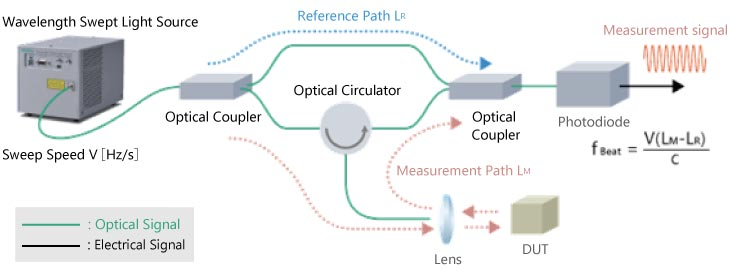The following figure shows an example of OFDR measurement of a plastic model steam train.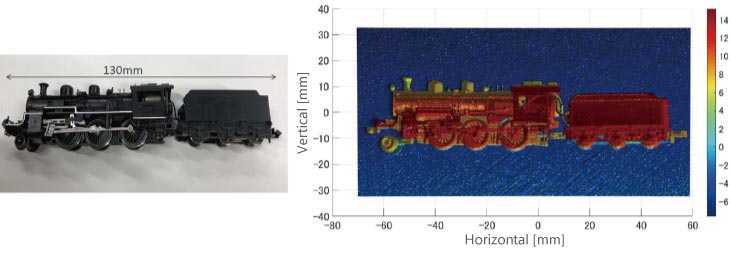## Opaque Body Thickness Measurement Example

Generally, optical length measurement methods cannot measure distance to the measurement point if there is no light refracted or dispersed from that point. Consequently, measurement of opaque bodies that do not transmit light cannot measure the distance from the back to front surfaces of the body, or in other words cannot measure thickness. The next part introduces a method for resolving this issue.

By using our high-coherence wavelength swept light source, the OFDR interferometer and interference-signal analysis section can be used as one to configure multiple laser-light output ports.

The following figure shows an example with three ports. If the lengths of the optical fibers at each port are preset so that the distances to the DUT do not overlap, it is possible to measure each distance L0, L1, and L2 from the output lens to the DUT as shown in the graph in the figure.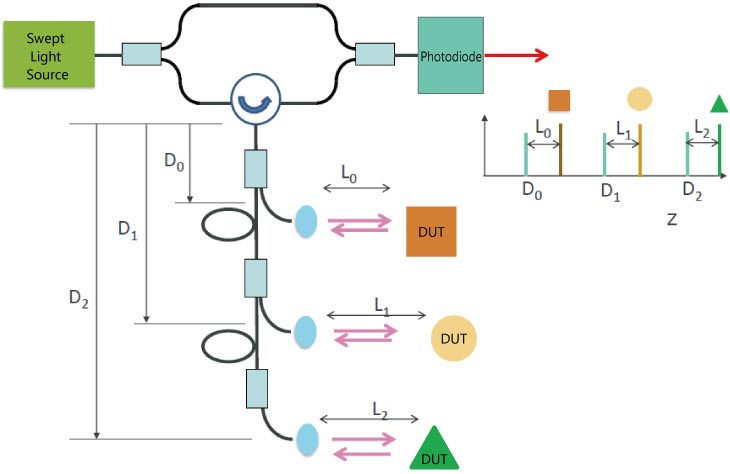The thickness of opaque bodies can be measured by applying this method. As shown in the following figure, arranging the laser light output ports to face each other and pre-measuring the distance between these facing ports as LAll before inserting the DUT between the two ports to measure the distance from the left port to the DUT as LLeft and the distance from the right port to the DUT as LRight makes it possible to calculate the thickness T of the opaque DUT as T = LAll — (LLeft + LRight).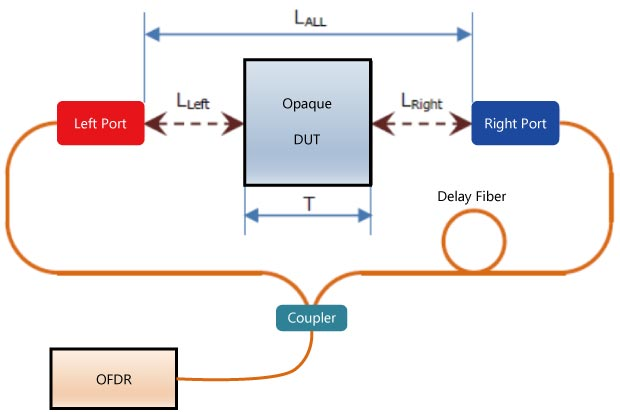The following figure shows an example using this method to measure an alumite processes metal block. The red and blue straight lines show the measurement location using OFDR. The bottom part of this figure shows the measurement results as a cross section of the measured line calculated from the measured distances from the two ports.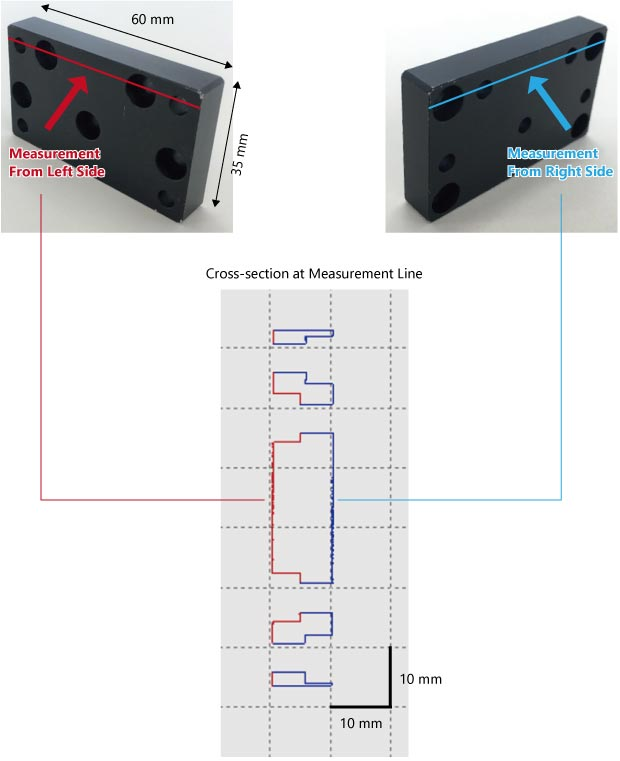## DUT Internal Structure Measurement Example

Optical Coherence Tomography (OCT) is an application of OFDR technology. OCT can measure semitranslucent objects that are able to transmit some light; it is a technology for capturing cross-sectional images of internal structure using internally reflected and dispersed light rather than light from the surfaces. Depth measurement of the internal structure is limited to within the range of the light-source coherence length as well as by capturing sufficient returning the light necessary for measurement.

The following figure shows an example of measurement of cushioning material filled with an air bubble structure using our wavelength swept light source. From this example it is clear that the internal air bubble structure of the cushion material can be measured by OCT. Since our light source has a long coherence length, lengths of about 1 meter can be measured, but in this cushioning material example, the effect of light absorption and scattering by the material limits the measurable depth to about 5 mm.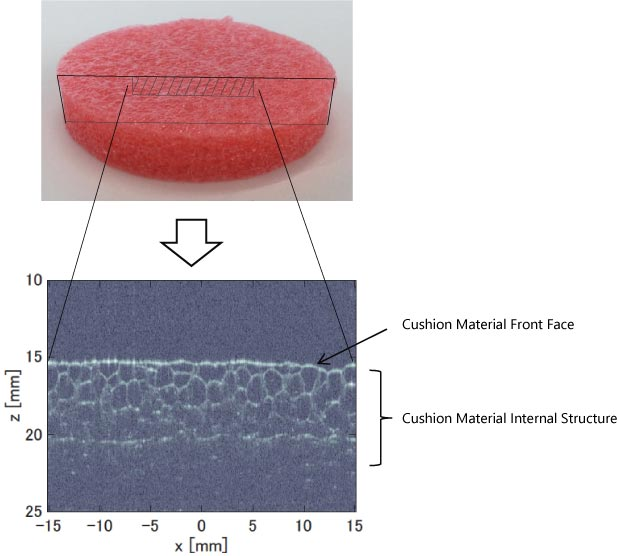The examples introduced in this lecture explain how OFDR measurement using our wavelength swept light source supports measurement of DUT surface shape, opaque-DUT thickness, and internal structure.